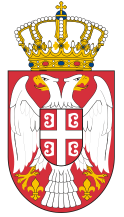Dokument se učitava

# MechanicsCourse code: 140107 | 6 ECTS credits

Basic information
Level of Studies: Undergraduate applied studies
Year of Study: 1
Semester: 2
Requirements:
Goal: The objective of this course is to introduce students to the basic laws in mechanics, in order to help them in solving problems of controlling mechanical systems.
Outcome: After passing the exam, the student is ready to successfully listen to professional subjects in the field of robotics and industrial automation and solve simpler problems from statics and dynamics of mechanical systems.
Contents of the course
Theoretical instruction:
1. Introduction. Areas in Mechanics.
2. Statics. The notion of force. Loe of Action and Reaction
3. Balance of the forces that cut at one point. Balance of the Plate Force System
4. Balance of the spatial Force System.
5. Kinematics. Position and movement.
6. Coordinate systems (DeCart system, cylinder system, natural way of displaying motion).
7. Speed ​​and acceleration.
8. Dynamics of the material point. Force and motion - Newton's law (vector and in different coordinate systems).
9. Oscillatory motion.
10. Power and energy.
11. Rotational movement. Angular velocity and acceleration.
12. Dynamic rotation equation. Kinetic energy at rotation.
13. General theorems of dynamics (maintenance laws). The theorem on changing the amount of motion. Theorem on the change of the momentum of motion.
14. The kinetic energy change theory.
15. Crash theory
Practical instruction (Problem solving sessions/Lab work/Practical training):
Textbooks and References
1. D.Mikičić, D.Popović, Mehanika, Građevinska knjiga, Beograd, 1993
Number of active classes (weekly)
Lectures: 3
Practical classes: 2
Other types of classes: 0
Grading (maximum number of points: 100)
Pre-exam obligations
Points
activities during lectures
0
activities on practial excersises
0
seminary work
20
colloquium
40
Final exam
Points
Written exam
40
Oral exam
0© 2019 School of Electrical and Computer Engineering of Applied Studies, Belgrade
Vojvode Stepe 283, office@viser.edu.rs, +381 11 2471 099